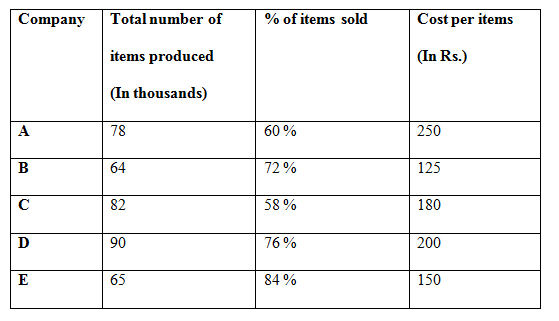# SBI PO Prelims Quantitative Aptitude Questions 2019 – Day 22

SBI PO 2019 Notification will be expected soon. It is one of the most expected recruitment among the banking aspirants. Every year the exam pattern for SBI PO has been changing. Depends upon the changing of exam pattern the questions are quite harder compare to the previous year. So the questions are in high level than the candidate’s assumption.

As per the latest trend, our IBPS Guide is providing the updated New Exam Pattern Quantitative Aptitude questions for SBI PO 2019 Day 22. Our Skilled experts were mounting the questions based on the aspirant’s needs. So candidates shall start your preparation and practice on daily basis with our SBI PO pattern quantitative aptitude questions 2019 day 22. Start your effective preparation from the right beginning to get success in upcoming SBI PO 2019.

[WpProQuiz 5429]

### Click Here for SBI PO Pre 2019 High-Quality Mocks Exactly on SBI Standard

Directions (1 – 5): What approximate value should come in the place of question mark (?) in the following questions?

1) (24.13)2 – (13.3)2 – (4338.17 + 179.75) ÷? = 156.27

a) 34

b) 18

c) 25

d) 42

e) 6

2) 5400.98 ÷ 19.81 × 29.97 =? ÷ 15.089 × 30.01

a) 4050

b) 3820

c) 3570

d) 4260

e) 4580

3) 59.85 % of 1548 + 43.899 % of 1049 = 20 % of ?

a) 6780

b) 6530

c) 6960

d) 6120

e) 5870

4) 49.72 × 1.98 × (45.09 – 18.21) + 168 ÷ 4 ÷ 1.81 – (9.87)2 = ?

a) 3560

b) 3130

c) 2850

d) 2620

e) 3340

5) (48.92)3 ÷ ((49.13) × 6.78)3 × (2400.66) = 7?

a) 3

b) 2

c) 1

d) 4

e) 5

Directions (6 – 10): Study the following graph carefully and answer the given question.

The following table shows the total number items produced by 5 different companies (In thousands) and the percentage of items sold and the cost per items.6) Total number of items sold by the company A is approximately what percentage of total number of items sold by the company C?

a) 98 %

b) 90 %

c) 80 %

d) 115 %

e) 65 %

7) Find the total cost earned by the company B and D together?

a) 1.728 Crore

b) 1.856 Crore

c) 1.642 Crore

d) 1.944 Crore

e) None of these

8) Find the ratio between the total number of items sold by the company B to that of company D?

a) 25: 36

b) 64: 95

c) 52: 77

d) 48: 69

e) None of these

9) Find the difference between the total cost earned by the company A to that of company E?

a) 0.351 Crore

b) 3.51 Crore

c) 0.0351 Crore

d) 35.1 lakhs

e) None of these

10) Total number of items produced by the company A and D together is approximately what percentage more/less than the total number of items produced by the company B and C together?

a) 25 % more

b) 20 % less

c) 15 % more

d) 15 % less

e) 20 % more

(24)2 – (13)2 – (4338 + 180) ÷ x = 156

576 – 169 – (4518/x) = 156

4518/x = 576 – 169 – 156

4518/x = 251

X = 4518/251 = 18

5400 ÷ 20 × 30 = x ÷ 15 × 30

5400/20 = x/15

X = (5400/20)*15 = 4050

60 % of 1550 + 44 % of 1050 = 20 % of x

(60/100)*1550 + (44/100)*1050 = (20/100)*x

930 + 462 = x/5

X = 1392*5 = 6960

50 × 2 × (45 – 18) + 168 ÷ 4 ÷ 2 – (10)2 = x

X = (50*2*27) + (168/8) – 100

X = 2700 + 21 – 100 = 2621

X = 2620

(49)3 ÷ (49 × 7)3 × (2401)2 = 7x

(72)3 ÷ (72 × 7)3 × 74 = 7x

76 ÷ (73)3 × 74 = 7x

76 – 9 + 4 = 7x

7x = 71

X = 1

Total number of items sold by the company A

= > 78*(60/100)

Total number of items sold by the company C

= > 82*(58/100)

Required % = {[78*(60/100)]/ [82*(58/100)]}*100 = 98 %

The total cost earned by the company B and D together

= > 64*(72/100)*125 + 90*(76/100)*200

= > (5760 + 13680) thousands

= > 1.944 Crore

The total number of items sold by the company B

= > 64*(72/100)

The total number of items sold by the company D

= > 90*(76/100)

Required ratio = [64*(72/100)]: [90*(76/100)]

= > 64: 95

The total cost earned by the company A

= > 78*(60/100)*250 = 11700 thousand

= > 1.17 Crore

The total cost earned by the company E

= > 65*(84/100)*150 = 8190 thousand

= > 0.819 Crore

Required difference = 1.17 – 0.819 = 0.351 Crore

Total number of items produced by the company A and D together

= > 78 + 90 = 168000

Total number of items produced by the company B and C together

= > 64 + 82 = 146000

Required % = [(168000 – 146000)/146000]*100 = 15 % more